www.batmath.it

### Examples and further techniques

Through the examples in this page we show some of the most common techniques used in the calculation of limits.

1.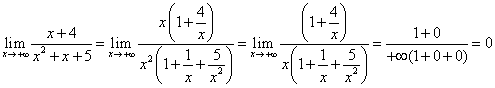. Use this technique when searching the limit of a rational function with x→±∞.

2.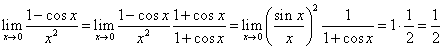.

3.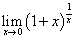. Change the variable from x to t=1/x. As x tends to zero, t tends to infinity. Thus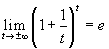.

4.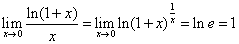5.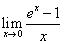. Change the variable from x to ex-1=t. As x tends to zero, t tends also to zero. The limit transforms in the reciprocal of limit 4: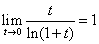.

6.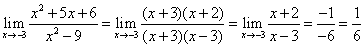. This technique often works in the case of a rational function in the form 0/0.

7.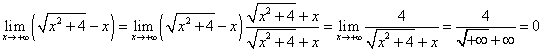.

8.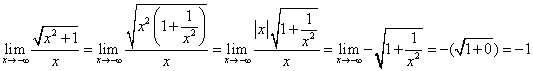.

9.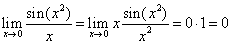. Here we have implicitly used a substitution x2=t. As x tends to zero, also t tends to zero. We can treat many other cases in the same way: usually the substitution will not be written explicitly. See the following example.

10.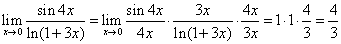.

first published on march 26 2002 - last updated on september 01 2003